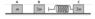# Momentum and Conservation of energy

wcjy
Homework Statement:
Objects A, B, and C with masses m, 2m, and 2m respectively, slide on a horizontal frictionless surface and object C is connected with a massless spring with spring constant k. Object A travels at a velocity v to the right and objects B and C are stationary initially. During the collisions between A, B, and C, show that the maximum change in the length of the spring is sqrt (4mv^2/9k). Note that the collision between A and B is elastic.
Relevant Equations:
EPE = 0.5kx^2
When A hits B,
COLM
mV = -mVa + 2mVb
V = 2Vb - Va
COKE
0.5mv^2 = 0.5mVa^2 + 0.5(2m)Vb^2
V^2 = Va^2 + 2Vb^2

When B hits C
COLM
2mVb=4mVc
Vc = 0.5Vb
COE
0.5(2m)Vb^2 = 0.5kx^2 +0.5(4m)Vc^2
sub Vc = 0.5b
mVb^2 = KX^2

After that I am stuck, cause i can't find V in terms of Vb only

#### Attachments

•phpQvI4xA.png
2.6 KB · Views: 75

Hello @wcjy ,You have $$v = 2v_b - v_a \qquad \& \qquad v^2 = v_a^2 + 2v_b^2$$ which should be enough to get ##v_b##.
Hello @wcjy ,You have $$v = 2v_b - v_a \qquad \& \qquad v^2 = v_a^2 + 2v_b^2$$ which should be enough to get ##v_b##.